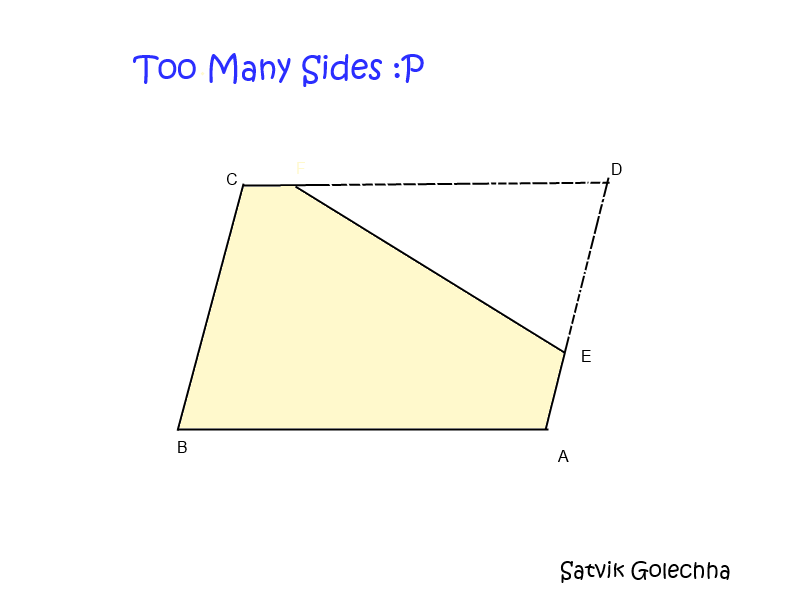# Too Many Sides, Too Little InformationAn equiangular quadrilateral ABCD has integral sides. A straight cut is made, which divides it into a triangle with integer sides, and a pentagon with a perimeter of 108 units. Given that four of the sides of the pentagon are 24, 18, 17 and 10 units, what is the area of the triangle (in square units)?

This question was given to me by a teacher.

×